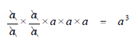## Some other Rules Assignment Help

Assignment Help: >> Basic Rules for Exponents - Some other Rules

Some other Rules:

Rule 3: While dividing two exponential numbers, subtract the powers.

am/an = am-n

Example:

a5/a2 = a×a×a×a×a/a×a  =Rule 4: Any exponential number is equal to one while divided by itself.

an/an  =1

Rule 5: To raise a product to a power so raises each factor to that power.

(ab)n  = anbn

That arises from the associative law for multiplication, that is, order of multiplication does not alter the product.

Example:

(ab)2  = (a x b) x (a x b) = (a x a) (b x b) = a2  x b2

If doubt exists in the student's mind, try multiplying (2 x 3)2 out in different orders.  All orders will yield 36.

Rule 6: For raise a quotient to a power, raise both the numerator and denominator to that power.

(a/b)n = an/bn

Example:

To demonstrate this, consider

(3/2)2 = 1.52 =2.25 = 2(1/4) = 9/4

But 32/22 = 9/4 the same value